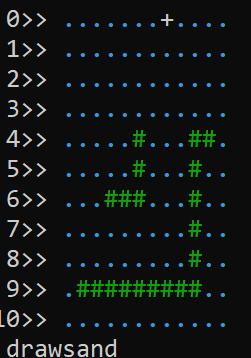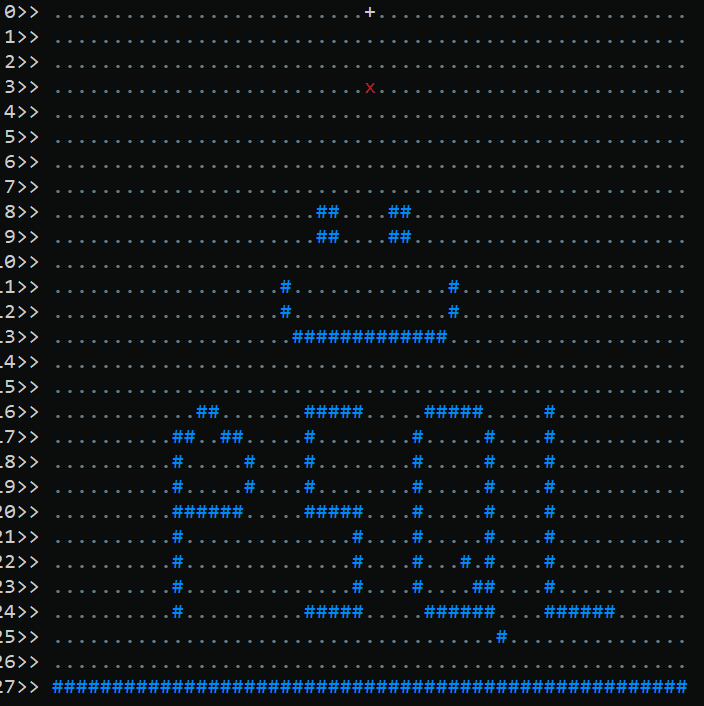# How to Solve Advent of Code 2022 Using Postgres - Day 14

## Disclaimer

This article will contain spoilers both on how I solved 2022 Day 14's challenge "Regolith Reservoir" using SQL, as well as general ideas on how to approach the problem. I recommend trying to solve it yourself first, using your favorite language. This article is delayed from the actual puzzle's release. Also note that my solutions are seldom going to be the "best" solutions - they were solved as quickly as possible, and these articles will show my first solutions, with some minor reformatting and cleaning up.

## Hands on Tutorial

We've also loaded a tutorial for Day 14's challenge if you want to try it with a pre-loaded data set.

## AOC Day 14

For this challenge, there are grains of sand falling from the top of a chamber. These grains fall onto various rock formations, and pile up on them, until they eventually spill onto the floor. We are tasked with figuring out how many grains fall before they spill, given dimensions about the rock formations. We are going to use a handful of tech for this, including:

• file_fdw to turn our input file into a table
• A sequence to enable simple counting of items
• The string_to_table() and split_part() functions to help us transform the text input to usable parts
• The lag() function to let us work with two rows at once
• The generate_series() function to allow population of a table in a certain range of values

``````CREATE EXTENSION if not exists file_fdw;

CREATE SERVER if not exists aoc2022 FOREIGN DATA WRAPPER file_fdw;

DROP SCHEMA if exists aoc_flow CASCADE;
CREATE SCHEMA aoc_flow;
SET search_path = aoc_flow;

DROP FOREIGN TABLE if exists aoc_day14;

CREATE FOREIGN TABLE aoc_day14 (line text)
SERVER aoc2022 options(filename '/tmp/aoc2022.day14.input');

DROP SEQUENCE if exists aoc;
CREATE SEQUENCE aoc;
``````

Our input file is a string of numbers denoting the edges of some (mostly U-shaped) rocks, and looks like this:

``````498,4 -> 498,6 -> 496,6
503,4 -> 502,4 -> 502,9 -> 494,9
``````

The directions indicate that those numbers map to something resembling this:

``````  4     5  5
9     0  0
4     0  3
0 ......+...
1 ..........
2 ..........
3 ..........
4 ....#...##
5 ....#...#.
6 ..###...#.
7 ........#.
8 ........#.
9 #########.
``````

Because we eventually want to get everything into a grid like the one above, let's make a simple table to hold each point and what it contains:

``````DROP TABLE if exists rockgrid;
CREATE UNLOGGED TABLE rockgrid (
row int,
col int,
item text
);
CREATE UNIQUE INDEX rockslot ON rockgrid(row,col);
``````

We want to break the input lines into separates rocks (one per line), and divide into the edges like so:

``````WITH x AS (SELECT nextval('aoc') AS rock, line FROM aoc_day14)
SELECT rock, string_to_table(line, ' -> ') AS points FROM x LIMIT 10;
``````
`````` rock | points
------+--------
1 | 498,4
1 | 498,6
1 | 496,6
2 | 503,4
2 | 502,4
2 | 502,9
2 | 494,9
(7 rows)
``````

This test input only has 7 rows, but the full data set has many more. The next step is to turn these numbers, which represent corners/vertices, into a full grid, where all the points between the vertices are represented. This is a little tricky, as there are a lot of numbers between the corners of the rock that need to be drawn. So we need to find every two vertices, then find a way to generate all the numbers between them. That is definitely a job for the `generate_series()` function. Because we also need to look at the current and the "previous" corner, we will use the window function `lag()` to be able to see both at once. For each pair of points, we will figure out the start and stop point in both grid directions (row and col), then use those numbers to fill in all the grid items between them. As we need those min and max entries to be generated and referenced for every point, we make sure they are called with `lateral`. Finally, we stick them all into our new table, using `distinct` to remove any duplicate entries:

``````WITH x AS (SELECT nextval('aoc') AS rock, line FROM aoc_day14)
,y AS (SELECT rock, string_to_table(line, ' -> ') AS
ypoint FROM x)
,z AS (SELECT *, split_part(ypoint, ',', 1)::int AS xx, split_part(ypoint, ',', 2)::int AS yy FROM y)
,c AS (SELECT *, lag(xx) over () AS lagxx, lag(yy) over() AS lagyy, lag(rock) over() AS lagrock FROM z)
,xrows AS (SELECT col, row FROM c
,LATERAL (SELECT row FROM generate_series(least(yy,lagyy),greatest(yy,lagyy)) row) i
,LATERAL (SELECT col FROM generate_series(least(xx,lagxx),greatest(xx,lagxx)) col) h
WHERE rock = lagrock
)
INSERT INTO rockgrid (row,col,item) SELECT distinct row,col,'#' FROM xrows;
``````

(Yes, that was a lot to take in!) The puzzle also suggests using a period to represent places without a rock, i.e. "air", so we can use an upsert ("insert or update") statements to populate the rest of our grid:

``````WITH minmax AS ( -- get the boundaries of our current grid
SELECT
min(row) AS minr, max(row) AS maxr,
min(col) AS minc, max(col) AS maxc
FROM rockgrid)
INSERT INTO rockgrid (row,col,item)
SELECT x,y,'.' FROM minmax, generate_series(0,maxr+1) AS x, generate_series(minc-1,maxc+1) AS y
ON CONFLICT DO NOTHING; -- ignore if this grid spot already has something

-- Specify the source of the grains of sand:
UPDATE rockgrid SET item = '+' WHERE row=0 AND col=500;
``````

As a final sanity check, let's make a quick visualization function so our human brains can better see what the data in the table looks like:

``````CREATE or replace FUNCTION drawsand(xrow int, xcol int, herechar cahr default 'x')
returns void language plpgsql as \$\$
DECLARE
myrec record; output text = ''; myitem text;
BEGIN
-- Slow things own slightly. Comment out for max speed
PERFORM pg_sleep(0.1);
-- For now, just hardcode the rows to view
FOR x in 0..27 LOOP
-- Add a newline for a clean start, then show the row number
output = output || format('%s%2s>> ', chr(10), x);
-- Show a sensible number of columns
FOR y IN 474..526 LOOP
-- See what is at this point
SELECT INTO myitem item FROM rockgrid WHERE row=x AND col=y;

-- If the item does not exist yet, treat it as "air"
IF myitem is null THEN myitem = '.'; END IF;

-- Output the current character, with a specific color
IF x=xrow AND y=xcol THEN -- falling sand
output = output || format(E'\033[31m%s\033[0m', myitem);
ELSIF '#' = myitem THEN --rock
output = output || format(E'\033[38;5;33m%s\033[0m', myitem);
ELSIF '.' = myitem THEN --air
output = output || format(E'\033[38;5;8m%s\033[0m', myitem);
ELSIF 'o' = myitem THEN -- stuck sand
output = output || format(E'\033[38;5;221m%s\033[0m', myitem);
ELSE -- source of sand
output = output || format('%s', myitem);
END IF;
END LOOP;
END LOOP;
-- Clear the screen, then write out the string we have built
RAISE NOTICE '% %', E'\033c', output;
return;
END;
\$\$;
``````

Using the testdata above, this is the result of `SELECT rockdraw(0,0)`:

`````` 0>> .......+....
1>> ............
2>> ............
3>> ............
4>> .....#...##.
5>> .....#...#..
6>> ...###...#..
7>> .........#..
8>> .........#..
9>> .#########..
10>> ............
``````

Now we can write a function to implement the steps of the challenge. We release a grain of sand that falls downward due to gravity, then goes left if possible, then right is possible, until it cannot move further. At that point, the next grain of sand falls. Our job is to figure out how many grains of sand fall before the they start falling off the "edge" of the grid. For the function, we'll have two versions: one that just solves the problem, and one that shows a graphical output as it does so. The latter does not scale, but it does look pretty for our test input:``````CREATE or replace FUNCTION sandflow(grains int, drawit bool default false)
returns int language plpgsql as \$\$
DECLARE
mincol int; maxcol int; maxrow int;
xrow int; xcol int; moved bool;
active_char char = 'x'; totalgrains int = 0;
BEGIN
SELECT INTO mincol,maxcol,maxrow
min(col),max(col),max(row) FROM sandgrid WHERE item = '#';
<<mainloop>> FOR x IN 1..grains LOOP
xrow = 0; xcol = 500; moved = false; totalgrains = totalgrains + 1;
<<grain>> LOOP
-- Can it go down? Future optimization: move down multiple steps
PERFORM 1 FROM sandgrid WHERE col=xcol AND row=xrow+1 AND item = '.';
IF found THEN xrow = xrow + 1; moved = true;
ELSE
-- Cannot go down, so try diagonal left
PERFORM 1 FROM sandgrid WHERE col=xcol-1 AND row=xrow+1 AND item = '.';
IF found THEN xrow = xrow + 1; xcol = xcol - 1; moved = true;
else
-- Cannot go diagonal left, so try diagonal right
PERFORM 1 FROM sandgrid WHERE col=xcol+1 AND row=xrow+1 AND item = '.';
IF found THEN xrow = xrow + 1; xcol = xcol + 1; moved = true;
ELSE exit; -- move to the next grain
END IF;
END IF;
END IF; -- end check down ok, then left/right
IF drawit THEN PERFORM drawsand(xrow,xcol,active_char); END IF;
CONTINUE grain; -- let this grain try and move again
END LOOP; -- <<grain>>
IF moved THEN
IF xrow > maxrow OR xcol=mincol OR xcol>maxcol THEN
active_char = '~'; -- indicating "forever falling"
IF drawit THEN continue; END IF;
return x-1;
END IF;
-- The sand grain has settled in place
UPDATE sandgrid SET item = 'o' WHERE row=xrow and col=xcol;
ELSE raise notice '(%,% at %) Cannot move at all', xrow, xcol, x;
exit mainloop;
END IF;
END LOOP;
END;
\$\$;
``````

I created a new input file for something larger then the test input above, but still smaller than the giant "actual" file the test gave. Plus, this one looks nice. Here it is:

``````484,24 -> 484,20 -> 489,20 -> 484,20 -> 484,17 -> 485,17
486,16 -> 487,16
488,17 -> 489,17
490,18 -> 490,19
499,16 -> 495,16 -> 495,20 -> 499,20 -> 499,24 -> 495,24
505,16 -> 509,16
510,17 -> 510,24 -> 509,23
509,24 -> 505,24
504,23 -> 504,17
511,25 -> 511,25
508,22 -> 508,22
515,16 -> 515,24 -> 520,24
493,11 -> 493,12
494,13 -> 506,13
507,12 -> 507,11
496,8 -> 497,9
502,8 -> 503,9
``````

Calling our new function with a lot of grains and a second argument of "false" shows the correct answer:

``````SELECT sandflow(5555, false);
``````
``````sandflow
----------
50
``````

For the second part of the challenge, the rules change a little bit. Now there is a "floor" of infinite dimensions, and we need to determine how many grains fall until things get clogged up and no more grains can fall. For this, we can use the exact same `sandflow` function, we just need to modify the map and add a floor. First, let's reset the grid and change any sand particles back to air:

``````UPDATE sandgrid SET item = '.' WHERE item = 'o';
``````

Now we need to extend the size of the grid by a good bit on both sides. We will add a row, expand the column in both directions, and add empty spots as needed:

``````WITH minmax AS (SELECT min(row) AS minr, max(row) AS maxr,
min(col) AS minc, max(col) AS maxc FROM sandgrid)
INSERT INTO sandgrid (row,col,item)
SELECT x,y,'.' FROM minmax, generate_series(minr,maxr+1) as x, generate_series(minc-25,minc+46) as y
ON CONFLICT DO NOTHING;
``````

Finally, we add our new "floor":

``````UPDATE sandgrid SET item = '#' WHERE row = (SELECT max(row) FROM sandgrid);
``````

Upon running, the total grains has jumped quite a bit:

``````SELECT sandflow(55555, false);
``````
``````NOTICE:  (0,500 at 543) Cannot move at all
sandflow
----------
543
(1 row)
``````

That's all for today's challenge! Stay tuned for more. I will leave you with the output of running the same function, but with a `true` argument, which adds some nice animation:Stay tuned for more days solving Advent of Code using Postgres!Written by

Greg Sabino Mullane

January 24, 2023 More by this author